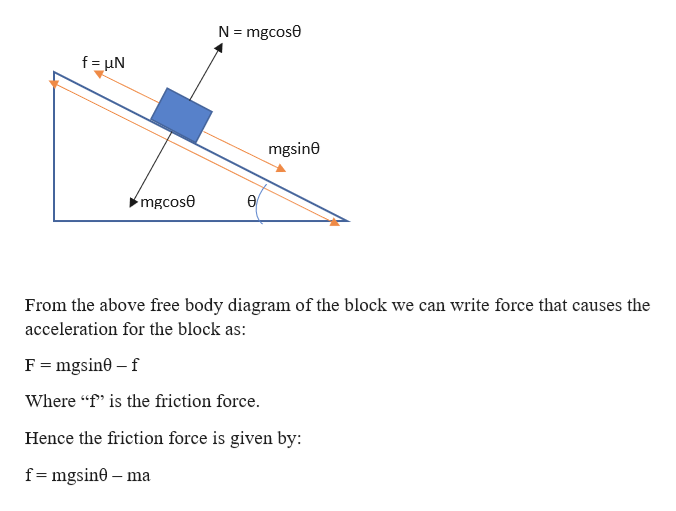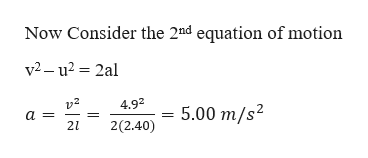# A 2.30 kg block slides down a frictionless incline from rest at the top (length = 2.40m, angle = 55°) and has a measured speed of 6.28 m/s at the bottom.  The block is then slid down a rough incline of same length and angle and found to achieve a speed of 4.90 m/s.  What is the best answer for the average frictional force experienced by the block while sliding down the second incline?

Question
35 views

A 2.30 kg block slides down a frictionless incline from rest at the top (length = 2.40m, angle = 55°) and has a measured speed of 6.28 m/s at the bottom.  The block is then slid down a rough incline of same length and angle and found to achieve a speed of 4.90 m/s.  What is the best answer for the average frictional force experienced by the block while sliding down the second incline?

check_circle

Step 1

Given information:

Mass of the block (m) = 2.30 kg

Length of the incline (l) = 2.40 m

Angle of inclination (θ) = 550

Initial velocity of block (u) = 0 m/s

Final velocity of block when the block is incline frictionless (v) = 6.28 m/s

Final velocity of block when the block is incline friction (vf) = 4.90 m/s

Step 2

Free body diagram:help_outlineImage TranscriptioncloseN mgcose f uN mgsine mgcose From the above free body diagram of the block we can write force that causes the acceleration for the block as: mgsin0 f Where " is the friction force. Hence the friction force is given by: f mgsin0 ma fullscreen
Step 3

We can find the acceleration of the block as the final velocity ...help_outlineImage TranscriptioncloseNow Consider the 2nd equation of motion v2-u2 2al v2 a = 4.92 5.00 m/s2 2(2.40) 21 | fullscreen

### Want to see the full answer?

See Solution

#### Want to see this answer and more?

Solutions are written by subject experts who are available 24/7. Questions are typically answered within 1 hour.*

See Solution
*Response times may vary by subject and question.
Tagged in
SciencePhysics

### Friction